# 密码学简介

• 单表替换加密
• 多表替换加密
• 奇奇怪怪的加密方式

• 对称密码，以 DES，AES，RC4 为代表。
• 非对称密码，以 RSA，椭圆曲线加密为代表。
• Hash，以 MD5，SHA1，SHA512 等为代表。
• 数字签名，以 RSA 签名，ElGamal 签名，DSA 签名为代表。

• 块加密
• 流加密

• 保密性
• 完整性
• 可用性
• 不可否认性

• 可汗学院公开课
• 深入浅出密码学——常用加密技术原理与应用

• 维基百科-密码学

# 古典密码简介

shannon的保密系统的通信理论发表前的密码都归为古典密码，古典密码编码方法归根结底主要有两种，即置换和代换。

### 置换密码

- 列置换 加密：将明文按固定长m分组，即每行m个字母，在密钥控制下按某一顺序交换列，最后按列优先的顺序依次读出，即产生了密文。 解密：逆过程。

- 周期置换 很大程度上同列置换，只不过加、解密时，在列交换后是按行优先的顺序向下进行。

### 代换

②乘法密码 A和B是有n个字母的字母表。?定义一个由A到B的映射：f:A→B 其中:(n,k)=1。 注意：只有(n,k)=1，才能正确解密。

③密钥词组代替密码 随机选一个词语，去掉其中的重复字母，写到矩阵的第一行，从明文字母表中去掉这第一行的字母，其余字母顺序写入矩阵。然后按列取出字母构成密文字母表。

- 多表代换密码 单表代替密码的安全性不高，一个原因是一个明文字母只由一个密文字母代替。可以利用频率分析来破译。故产生了更为安全的多表代换密码，即构造多个密文字母表，在密钥的控制下用以一系列代换表依次对明文消息的字母序列进行代换。

# 单表代换加密

• 学习常见的几种加密方式

• Ubuntu

• JPK

## [原理]

• 在密钥空间较小的情况下，采用暴力破解方式
• 在密文长度足够长的时候，使用词频分析

明文字母表：ABCDEFGHIJKLMNOPQRSTUVWXYZ



明文：THE QUICK BROWN FOX JUMPS OVER THE LAZY DOG



• 偏移量为 10：Avocat （A→K）
• 偏移量为 13： ROT13，ROT13是它自己本身的逆反，也就是说，要还原ROT13，套用加密同样的算法即可得，故同样的操作可用再加密与解密。
• 偏移量为 -5：Cassis （K 6）
• 偏移量为 -6：Cassette （K 7）

## Atbash Cipher

### 原理

明文：A B C D E F G H I J K L M N O P Q R S T U V W X Y Z



## 简单替换密码

### 原理

明文字母 : abcdefghijklmnopqrstuvwxyz



a 对应 p，d 对应 h，以此类推。

## 仿射密码

### 原理

•  表示明文按照某种编码得到的数字
•    互质
•  是编码系统中字母的数目。

## [步骤]

#### 凯撒密码

明文：s0a6u3u1s0bv1a



1. 遍历 26 个偏移量，适用于普遍情况
2. 利用词频分析，适用于密文较长的情况。

• JPK，可解带密钥与不带密钥
• 凯撒密码在线解密工具

#### Atbash Cipher

明文：the quick brown fox jumps over the lazy dog



#### 简单替换密码

明文：the quick brown fox jumps over the lazy dog



• 词频分析破解工具

#### 仿射密码

x055813428157417
83333487328184883432893
877222121822517215

877222121822517215
0-21-21294273-126210294-63189-126147
055813428157417

ϕ(26)=ϕ(2)×ϕ(13)=12

12×26=312

y1=(ax1+b)(mod26)y2=(ax2+b)(mod26)

y1y2=a(x1x2)(mod26)

import sys
key = '****CENSORED***************'
flag = 'TWCTF{*******CENSORED********}'

if len(key) % 2 == 1:
print("Key Length Error")
sys.exit(1)

n = len(key) / 2
encrypted = ''
for c in flag:
c = ord(c)
for a, b in zip(key[0:n], key[n:2*n]):
c = (ord(a) * c + ord(b)) % 251
encrypted += '%02x' % c

print encrypted


c1=a1c+b1c2=a2c1+b2=a1a2c+a2b1c+b2=kc+d

805eed80cbbccb94c36413275780ec94a857dfec8da8ca94a8c313a8ccf9

import gmpy

key = '****CENSORED****************'
flag = 'TWCTF{*******CENSORED********}'

f = open('encrypted', 'r')
encrypted = [int(data[i:i + 2], 16) for i in range(0, len(data), 2)]
plaindelta = ord(flag) - ord(flag)
cipherdalte = encrypted - encrypted
a = gmpy.invert(plaindelta, 251) * cipherdalte % 251
b = (encrypted - a * ord(flag)) % 251
a_inv = gmpy.invert(a, 251)
result = ""
for c in encrypted:
result += chr((c - b) * a_inv % 251)
print result


shell
➜  TWCTF2016-super_express git:(master) ✗ python exploit.py
TWCTF{Faster_Than_Shinkansen!}


# 多表代换加密

### 原理

Playfair 密码（Playfair cipher or Playfair square）是一种替换密码，1854 年由英国人查尔斯·惠斯通（Charles Wheatstone）发明，基本算法如下：

1. 选取一串英文字母，除去重复出现的字母，将剩下的字母逐个逐个加入 5 × 5 的矩阵内，剩下的空间由未加入的英文字母依 a-z 的顺序加入。注意，将 q 去除，或将 i 和 j 视作同一字。
2. 将要加密的明文分成两个一组。若组内的字母相同，将 X（或 Q）加到该组的第一个字母后，重新分组。若剩下一个字，也加入 X 。
3. 在每组中，找出两个字母在矩阵中的地方。
• 若两个字母不同行也不同列，在矩阵中找出另外两个字母（第一个字母对应行优先），使这四个字母成为一个长方形的四个角。
• 若两个字母同行，取这两个字母右方的字母（若字母在最右方则取最左方的字母）。
• 若两个字母同列，取这两个字母下方的字母（若字母在最下方则取最上方的字母）。

P L A Y F
I R E X M
B C D G H
K N O Q S
T U V W Z


HI DE TH EG OL DI NT HE TR EX ES TU MP


BM OD ZB XD NA BE KU DM UI XM MO UV IF


• CAP4

### 原理

Polybius密码又称为棋盘密码，其一般是将给定的明文加密为两两组合的数字，其常用密码表

12345
1ABCDE
2FGHI/JK
3LMNOP
4QRSTU
5VWXYZ

Abtalp
Ddhozk
Fqfvsn
Ggjcux
Xmrewy

A D F G X 的由来：

1918 年，第一次世界大战将要结束时，法军截获了一份德军电报，电文中的所有单词都由 A、D、F、G、X 五个字母拼成，因此被称为 ADFGX 密码。ADFGX 密码是 1918 年 3 月由德军上校 Fritz Nebel 发明的，是结合了 Polybius 密码和置换密码的双重加密方案。

• CrypTool

## Vigenere 维吉尼亚密码

### 原理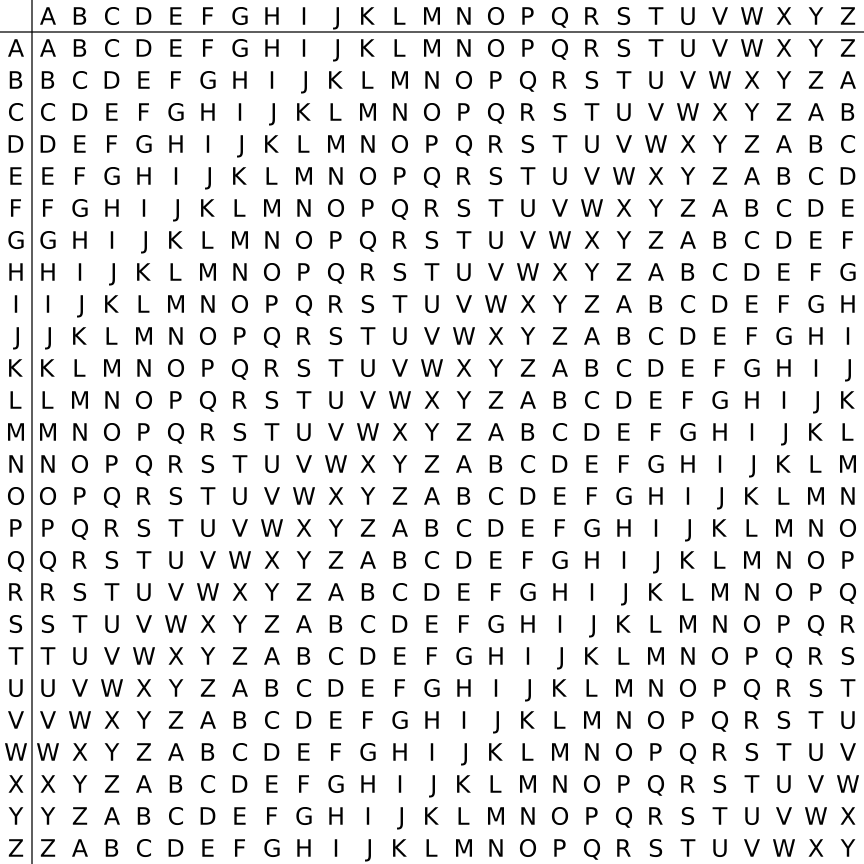明文：come greatwall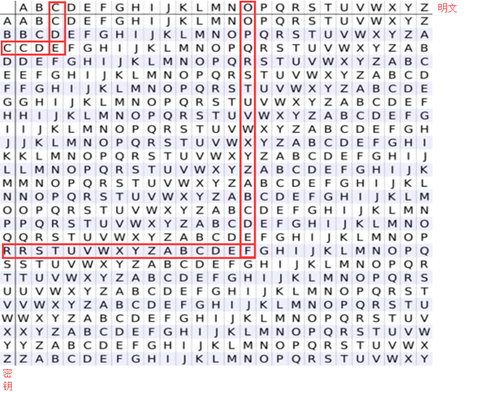明文：come greatwall



### 破解

密钥：ABCDEF AB CDEFA BCD EFABCDEFABCD



密钥：ABCDAB CD ABCDA BCD ABCDABCDABCD



密文：DYDUXRMHTVDVNQDQNWDYDUXRMHARTJGWNQD


### 工具

• 已知密钥
• Python 的 pycipher 库
• 在线解密 Vigenère cipher
• CAP4
• 未知密钥
• Vigenère Cipher Codebreaker
• Vigenere Solver, 不够完善。

## Nihilist

### 原理

Nihilist密码又称关键字密码：明文 + 关键字 = 密文。以关键字 helloworld 为例。

12345
1helow
2rdabc
3fgi / jkm
4npqst
5uvxyz

a -> M[2,3] -> 23
t -> M[4,5] -> 45


23 -> M[2,3] -> a
45 -> M[4,5] -> t


• 纯数字
• 只包含 1 到 5
• 密文长度偶数。

## Hill

### 原理

明文：ACT


0219$\left[\begin{array}{c}0\\ 2\\ 19\end{array}\right]$

6241131610201715$\left[\begin{array}{ccc}6& 24& 1\\ 13& 16& 10\\ 20& 17& 15\end{array}\right]$

624113161020171502196722231915147mod26$\left[\begin{array}{ccc}6& 24& 1\\ 13& 16& 10\\ 20& 17& 15\end{array}\right]\left[\begin{array}{c}0\\ 2\\ 19\end{array}\right]\equiv \left[\begin{array}{c}67\\ 222\\ 319\end{array}\right]\equiv \left[\begin{array}{c}15\\ 14\\ 7\end{array}\right]mod26$

密文：POH


• CAP4
• Cryptool

### 例子

1 4 7
2 5 8
3 6 10


## AutokeyCipher

### 原理

明文：THE QUICK BROWN FOX JUMPS OVER THE LAZY DOG



CULTURE THE QUICK BROWN FOX JUMPS OVER THE


VBP JOZGD IVEQV HYY AIICX CSNL FWW ZVDP WVK


### 工具

• 已知关键词
• Python 的 pycipher 库

# 其他类型加密

## 培根密码

### 原理

aAAAAAgAABBAnABBAAtBAABA
bAAAABhAABBBoABBABu-vBAABB
cAAABAi-jABAAApABBBAwBABAA
dAAABBkABAABqABBBBxBABAB
eAABAAlABABArBAAAAyBABBA
fAABABmABABBsBAAABzBABBB

To encode a message each letter of the plaintext is replaced by a group of five of the letters 'A' or 'B'.

• 只有两种字符
• 每一段的长度为 5
• 加密内容会有特殊的字体之分，亦或者大小写之分。

• 培根密码在线解密工具

## 栅栏密码

### 原理

明文：THERE IS A CIPHER


THEREISACIPHER


TH ER EI SA CI PH ER


TEESCPE
HRIAIHR


TEESCPEHRIAIHR


THEREIS ACIPHER


TAHCEIRPEHIESR


## 曲路密码

### 原理

明文：The quick brown fox jumps over the lazy dog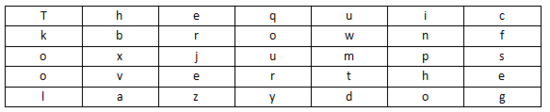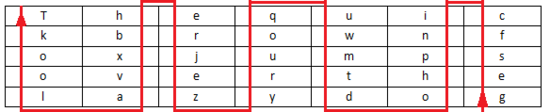密文：gesfc inpho dtmwu qoury zejre hbxva lookT


## 列移位加密

### 原理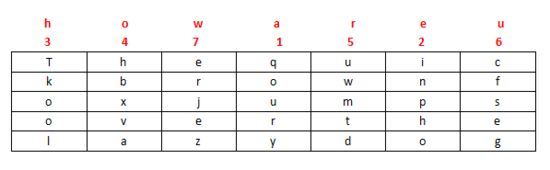## 01248 密码

• 只有 0，1，2，4，8

### 例子

8842101220480224404014224202480122

884218+8+4+2+1=23W
1221+2+2=5E
484+8=12L
22442+2+4+4=12L
44D
1422421+4+2+2+4+2=15O
2482+4+8=14N
1221+2+2=5E

## JSFuck

### 原理

JSFuck 可以只用 6 个字符 []()!+ 来编写 JavaScript 程序。比如我们想用 JSFuck 来实现 alert(1) 代码如下

[][(![]+[])[+[[+[]]]]+([][[]]+[])[+[[!+[]+!+[]+!+[]+!+[]+!+[]]]]+(![]+[])[+[[!+[]+!+[]]]]+(!![]+[])[+[[+[]]]]+(!![]+[])[+[[!+[]+!+[]+!+[]]]]+(!![]+[])[+[[+!+[]]]]][([][(![]+[])[+[[+[]]]]+([][[]]+[])[+[[!+[]+!+[]+!+[]+!+[]+!+[]]]]+(![]+[])[+[[!+[]+!+[]]]]+(!![]+[])[+[[+[]]]]+(!![]+[])[+[[!+[]+!+[]+!+[]]]]+(!![]+[])[+[[+!+[]]]]]+[])[+[[!+[]+!+[]+!+[]]]]+([][(![]+[])[+[[+[]]]]+([][[]]+[])[+[[!+[]+!+[]+!+[]+!+[]+!+[]]]]+(![]+[])[+[[!+[]+!+[]]]]+(!![]+[])[+[[+[]]]]+(!![]+[])[+[[!+[]+!+[]+!+[]]]]+(!![]+[])[+[[+!+[]]]]]+[])[+[[!+[]+!+[]+!+[]+!+[]+!+[]+!+[]]]]+([][[]]+[])[+[[+!+[]]]]+(![]+[])[+[[!+[]+!+[]+!+[]]]]+(!![]+[])[+[[+[]]]]+(!![]+[])[+[[+!+[]]]]+([][[]]+[])[+[[+[]]]]+([][(![]+[])[+[[+[]]]]+([][[]]+[])[+[[!+[]+!+[]+!+[]+!+[]+!+[]]]]+(![]+[])[+[[!+[]+!+[]]]]+(!![]+[])[+[[+[]]]]+(!![]+[])[+[[!+[]+!+[]+!+[]]]]+(!![]+[])[+[[+!+[]]]]]+[])[+[[!+[]+!+[]+!+[]]]]+(!![]+[])[+[[+[]]]]+([][(![]+[])[+[[+[]]]]+([][[]]+[])[+[[!+[]+!+[]+!+[]+!+[]+!+[]]]]+(![]+[])[+[[!+[]+!+[]]]]+(!![]+[])[+[[+[]]]]+(!![]+[])[+[[!+[]+!+[]+!+[]]]]+(!![]+[])[+[[+!+[]]]]]+[])[+[[!+[]+!+[]+!+[]+!+[]+!+[]+!+[]]]]+(!![]+[])[+[[+!+[]]]]]((![]+[])[+[[+!+[]]]]+(![]+[])[+[[!+[]+!+[]]]]+(!![]+[])[+[[!+[]+!+[]+!+[]]]]+(!![]+[])[+[[+!+[]]]]+(!![]+[])[+[[+[]]]]+([][(![]+[])[+[[+[]]]]+([][[]]+[])[+[[!+[]+!+[]+!+[]+!+[]+!+[]]]]+(![]+[])[+[[!+[]+!+[]]]]+(!![]+[])[+[[+[]]]]+(!![]+[])[+[[!+[]+!+[]+!+[]]]]+(!![]+[])[+[[+!+[]]]]]+[])[+[[+!+[]]]+[[!+[]+!+[]+!+[]+!+[]+!+[]]]]+[+!+[]]+([][(![]+[])[+[[+[]]]]+([][[]]+[])[+[[!+[]+!+[]+!+[]+!+[]+!+[]]]]+(![]+[])[+[[!+[]+!+[]]]]+(!![]+[])[+[[+[]]]]+(!![]+[])[+[[!+[]+!+[]+!+[]]]]+(!![]+[])[+[[+!+[]]]]]+[])[+[[+!+[]]]+[[!+[]+!+[]+!+[]+!+[]+!+[]+!+[]]]])()


false       =>  ![]
true        =>  !![]
undefined   =>  [][[]]
NaN         =>  +[![]]
0           =>  +[]
1           =>  +!+[]
2           =>  !+[]+!+[]
10          =>  [+!+[]]+[+[]]
Array       =>  []
Number      =>  +[]
String      =>  []+[]
Boolean     =>  ![]
Function    =>  []["filter"]
eval        =>  []["filter"]["constructor"]( CODE )()
window      =>  []["filter"]["constructor"]("return this")()


### 工具

• JSFuck 在线加密网站

## BrainFuck

### 原理

Brainfuck，是一种极小化的计算机语言，它是由 Urban Müller 在 1993 年创建的。我们举一个例子，如果我们想要一个在屏幕上打印Hello World！，那么对应的程序如下。对于其中的原理，感兴趣的可以自行网上搜索。

++++++++++[>+++++++>++++++++++>+++>+<<<<-]
>++.>+.+++++++..+++.>++.<<+++++++++++++++.
>.+++.------.--------.>+.>.


### 工具

• Brainfuck在线解密工具

## 猪圈密码

### 原理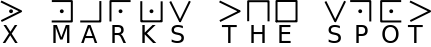## 舞动的小人密码

### 原理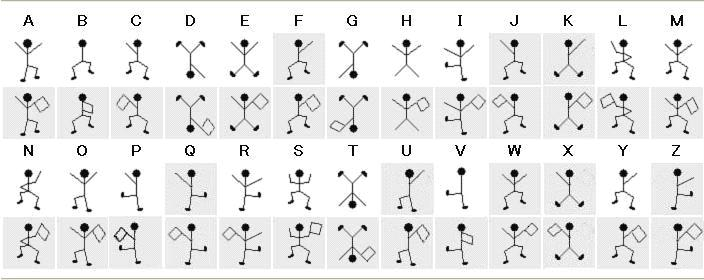## 键盘密码

### 手机键盘密码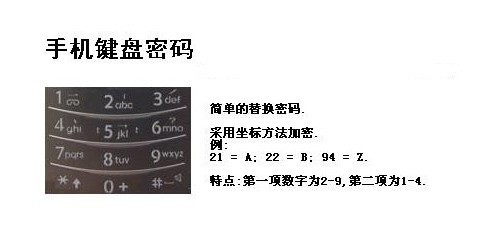### 电脑键盘棋盘¶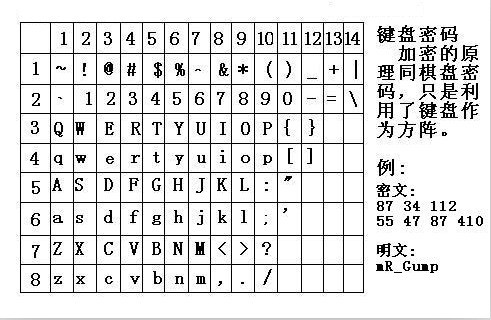### 电脑键盘坐标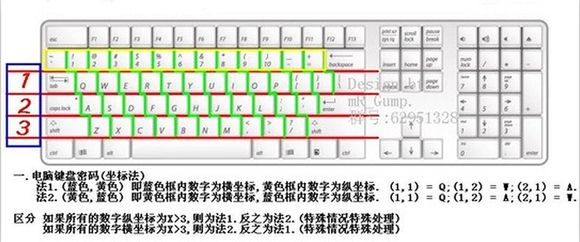### 电脑键盘 QWE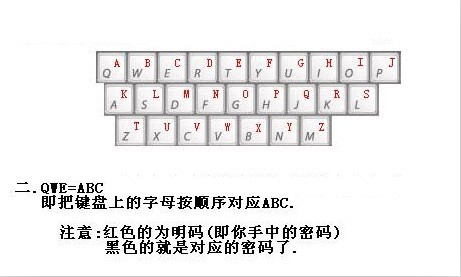### 2017年xman选拔赛——一二三，木头人

23731263111628163518122316391715262121

23-x

73-m

12-a

63-n

11-q

xman{hintisenough}


# 总结

• 已知密码，识别密码
• 未知密码，分析密码特性，利用暴力破解或者相应思路求解

• 加密方式判别
• 字符集判别
• 加密结果样子判别

#### 围在栅栏里的爱

-.- .. --.- .-.. .-- - ..-. -.-. --.- --. -. ... --- ---

flag格式：CTF{xxx}

#### 2017 SECCON Vigenere3d

# Vigenere3d.py
import sys
def _l(idx, s):
return s[idx:] + s[:idx]
def main(p, k1, k2):
s = "ABCDEFGHIJKLMNOPQRSTUVWXYZ0123456789abcdefghijklmnopqrstuvwxyz_{}"
t = [[_l((i+j) % len(s), s) for j in range(len(s))] for i in range(len(s))]
i1 = 0
i2 = 0
c = ""
for a in p:
c += t[s.find(a)][s.find(k1[i1])][s.find(k2[i2])]
i1 = (i1 + 1) % len(k1)
i2 = (i2 + 1) % len(k2)
return c
print main(sys.argv, sys.argv, sys.argv[::-1])

\$ python Vigenere3d.py SECCON{**************************} **************
POR4dnyTLHBfwbxAAZhe}}ocZR3Cxcftw9


t[i][j]=s[i+j:]+s[:i+j]t[i][k]=s[i+k:]+s[:i+k]$t\left[i\right]\left[j\right]=s\left[i+j:\right]+s\left[:i+j\right]\phantom{\rule{0ex}{0ex}}t\left[i\right]\left[k\right]=s\left[i+k:\right]+s\left[:i+k\right]$

t[i][j][k]$t\left[i\right]\left[j\right]\left[k\right]$  t[i][j]$t\left[i\right]\left[j\right]$ 中的第 k 个字符，t[i][k][j]$t\left[i\right]\left[k\right]\left[j\right]$  t[i][k]$t\left[i\right]\left[k\right]$ 中的第 j 个字符。无论是 i+j+k$i+j+k$ 是否超过 len(s) 两者都始终保持一致，即 t[i][j][k]=t[i][k][j]$t\left[i\right]\left[j\right]\left[k\right]=t\left[i\right]\left[k\right]\left[j\right]$

def get_key(plain, cipher):
s = "ABCDEFGHIJKLMNOPQRSTUVWXYZ0123456789abcdefghijklmnopqrstuvwxyz_{}"
t = [[_l((i + j) % len(s), s) for j in range(len(s))]
for i in range(len(s))]
i1 = 0
i2 = 0
key = ['*'] * 14
for i in range(len(plain)):
for i1 in range(len(s)):
for i2 in range(len(s)):
if t[s.find(plain[i])][s.find(s[i1])][s.find(s[i2])] == cipher[
i]:
key[i] = s[i1]
key[13 - i] = s[i2]
return ''.join(key)


def decrypt(cipher, k1, k2):
s = "ABCDEFGHIJKLMNOPQRSTUVWXYZ0123456789abcdefghijklmnopqrstuvwxyz_{}"
t = [[_l((i + j) % len(s), s) for j in range(len(s))]
for i in range(len(s))]
i1 = 0
i2 = 0
plain = ""
for a in cipher:
for i in range(len(s)):
if t[i][s.find(k1[i1])][s.find(k2[i2])] == a:
plain += s[i]
break
i1 = (i1 + 1) % len(k1)
i2 = (i2 + 1) % len(k2)
return plain


➜  2017_seccon_vigenere3d git:(master) python exp.py
SECCON{Welc0me_to_SECCON_CTF_2017}

# 对称加密简介

## 工作过程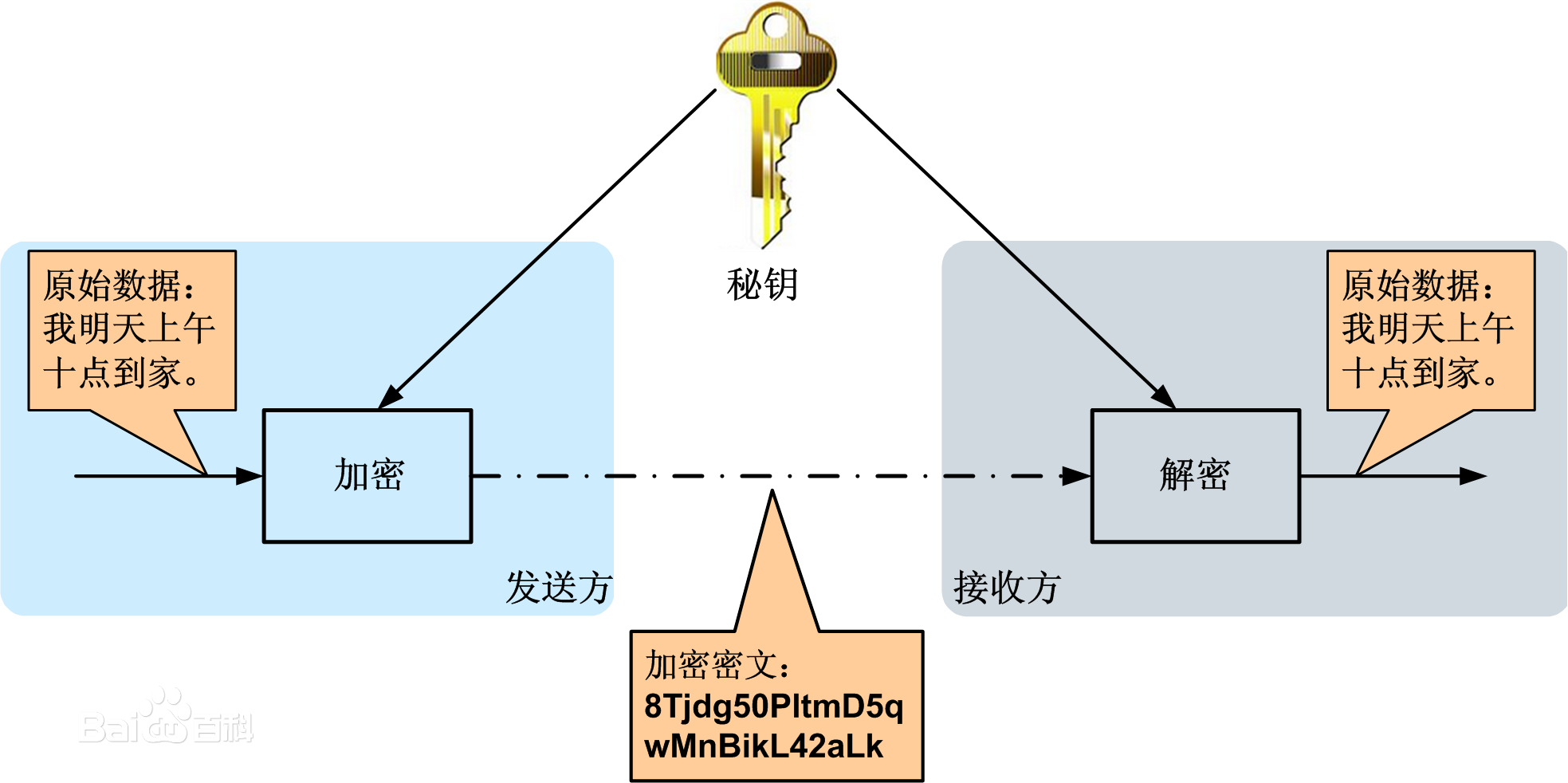## 特点

• 优点

• 不足之处
• 密钥传输问题：，由于对称加密的加密和解密使用的是同一个密钥，所以对称加密的安全性就不仅仅取决于加密算法本身的强度，更取决于密钥是否被安全的保管，因此加密者如何把密钥安全的传递到解密者手里，就成了对称加密面临的关键问题。（比如，客户端不能直接存储对称加密的密钥，因为被反编译之后，密钥就泄露了，数据安全性就得不到保障，所以实际中一般都是客户端向服务端请求对称加密的密钥，而且密钥还得通过非对称加密加密后再传输。）
• 密钥管理问题：随着密钥数量的增多，密钥的管理问题会逐渐显现出来。比如在加密用户的信息时，不能所有用户都用同一个密钥加密解密，否则，一旦一个用户密钥泄漏，就相当于泄露了所有用户的信息，因此需要为每一个用户单独的生成一个密钥并且管理，这样密钥管理的代价也会非常大。

• RC4加密
• DES加密
• 3DES加密
• AES加密

# ¶基本介绍¶

DES全称为Data Encryption Standard，是典型的块加密，其基本信息如下

• 使用64位密钥中的56位，剩余的8位要么丢弃，要么作为奇偶校验位
• 输入64位
• 输出64位。
• 明文经过16轮迭代得到密文。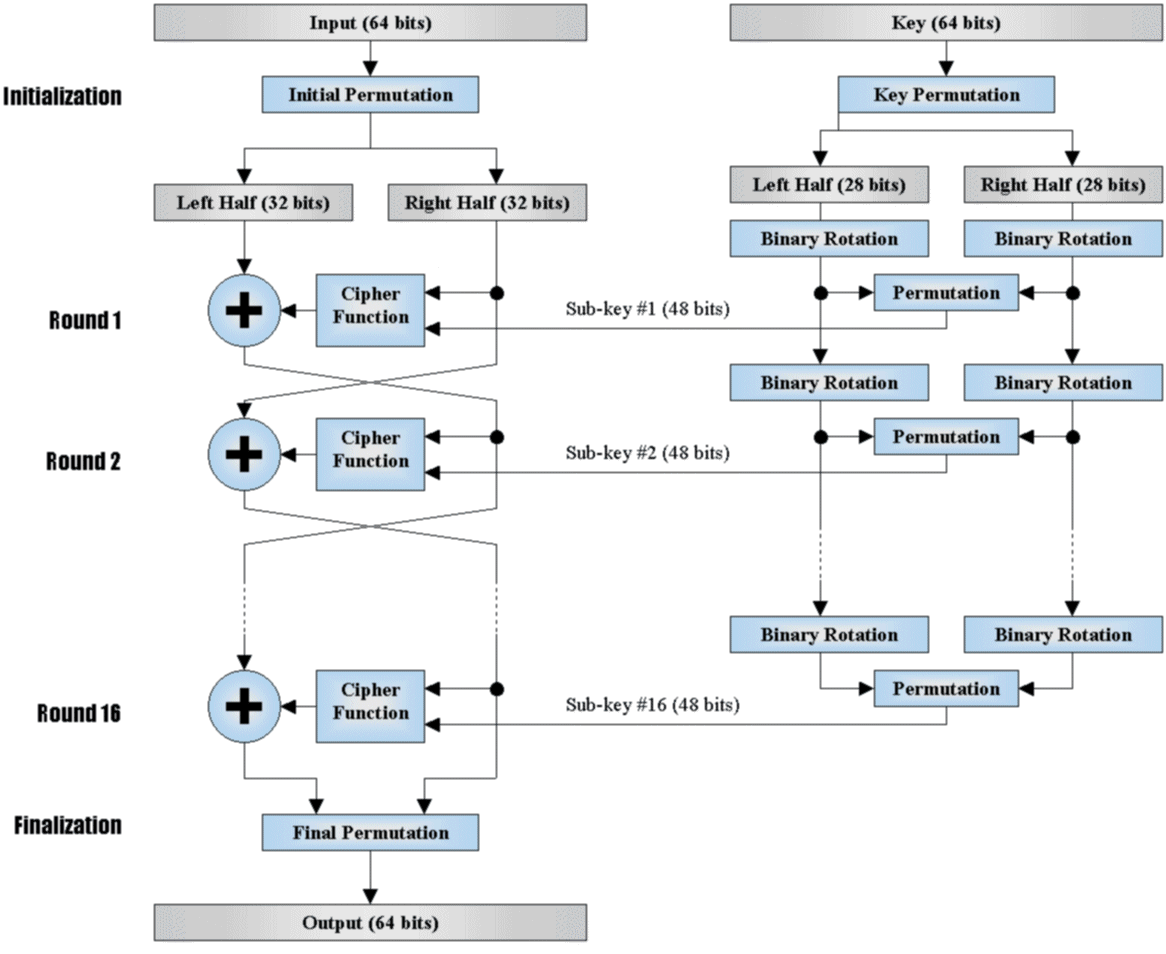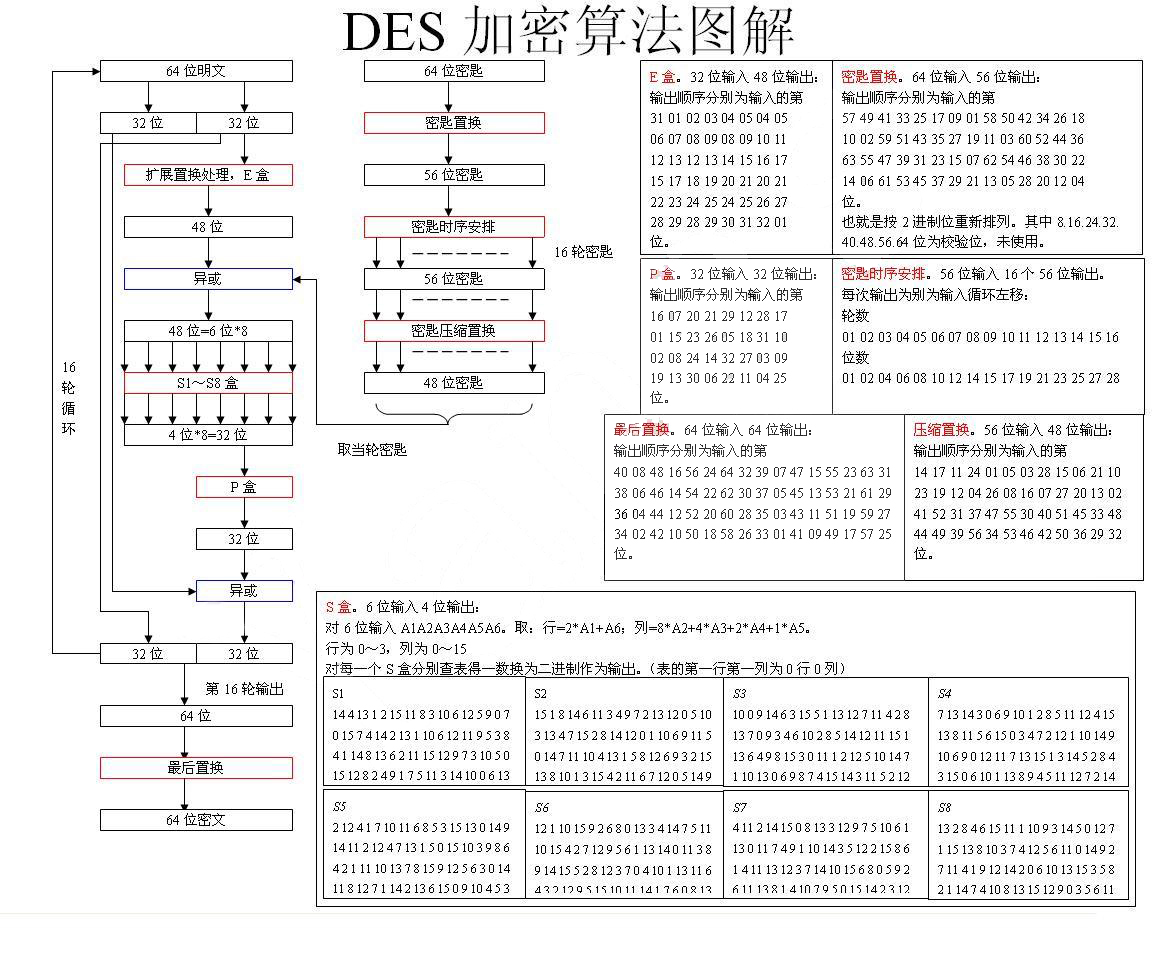• S盒的设计标准并未给出。

posted @ 2021-08-06 17:04  渗透测试中心  阅读(794)  评论(0编辑  收藏  举报